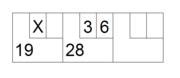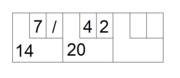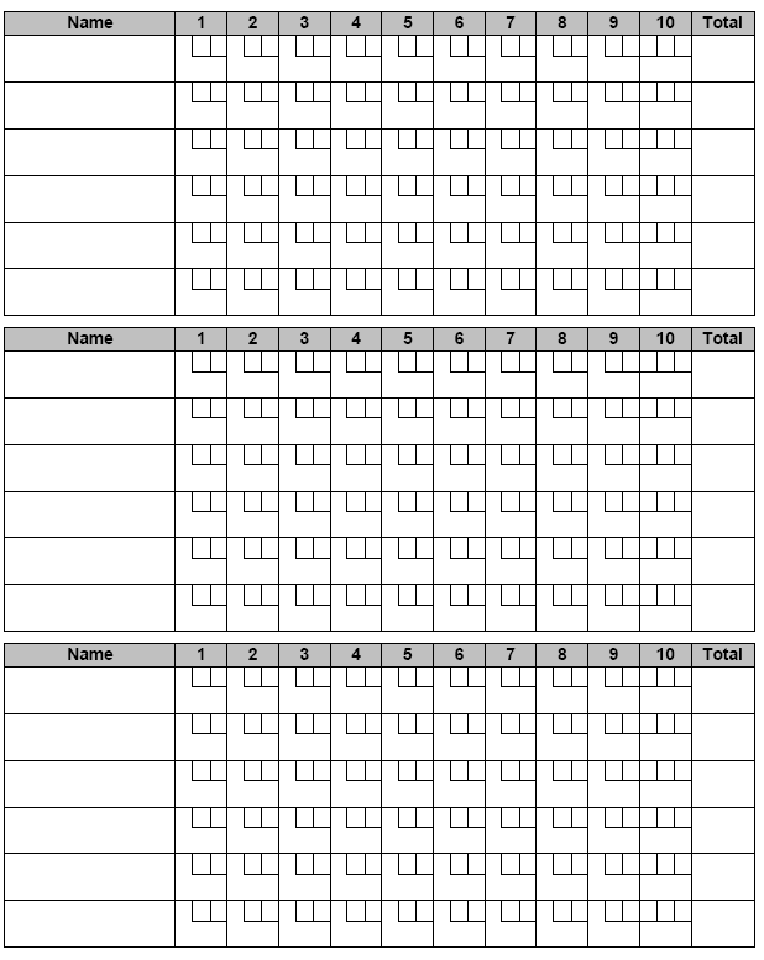Premier Ball Drilling in a Family Friendly Atmosphere!

Scoring

Home About Us / Contact Us / Honor Scores / LaborLinks / Pictures / Revivor / Specials / Shop Online / Testimonials / Tips / Videos

Spares / How To Bowl / Why / Scoring / Difference / Head Pin / Bowling Facts

In general, one point is scored for each pin that is knocked over. So if a player bowls over three pins with the first shot, then six with the second, the player would receive a total of nine points for that frame. If a player knocks down 9 pins with the first shot, but misses with the second, the player would also score nine. When a player fails to knock down all ten pins after their second ball it is known as an open frame. In the event that all ten pins are knocked over by a player in a single frame, bonuses are awarded. When all ten pins are knocked down with the first ball (called a strike and typically rendered as an “X” on a score sheet), a player is awarded ten points, plus a bonus of whatever is scored with the next two balls. In this way, the points scored for the two balls after the strike are counted twice.

Frame 1, ball 1: 10 pins (strike)

Frame 2, ball 1: 3 pins

Frame 2, ball 2: 6 pins

The total score from these throws is:

Frame one: 10 + (3 + 6) = 19

Frame two: 3 + 6 = 9

TOTAL = 28Two consecutive strikes are referred to as a “double.”

A double's pin fall is:

Frame 1, ball 1: 10 pins (Strike)

Frame 2, ball 1: 10 pins (Strike)

Frame 3, ball 1: 9 pins

Frame 3, ball 2: 0 pins (recorded as a dash '-' on the score sheet)

The total score from these throws is:

Frame one: 10 + (10 + 9) = 29

Frame two: 10 + (9 + 0) = 19

Frame three: 9 + 0 = 9

TOTAL = 57

Three strikes bowled consecutively are known as a “turkey” or “triple.”

A triple's pin fall is:

Frame 1, ball 1: 10 pins (Strike)

Frame 2, ball 1: 10 pins (Strike)

Frame 3, ball 1: 10 pins (Strike)

Frame 4, ball 1: 0 pins (Gutter ball)

Frame 4, ball 2: 9 pins

The total score from these throws is:

Frame one: 10 + (10 + 10) = 30

Frame two: 10 + (10 + 0) = 20

Frame three: 10 + (0 + 9) = 19

Frame four: 0 + 9 = 9

TOTAL = 78

A player who scores multiple strikes in succession would score like so:

Frame 1, ball 1: 10 pins (strike)

Frame 2, ball 1: 10 pins (strike)

Frame 3, ball 1: 4 pins

Frame 3, ball 2: 2 pins

The score from these throws are:

Frame one: 10 + (10 + 4) = 24

Frame two: 10 + (4 + 2) = 16

Frame three: 4 + 2 = 6

TOTAL = 46

The most points that can be scored in a single frame is 30 points (10 for the original strike, plus strikes in the two subsequent frames). A player who bowls a strike in the tenth (final) frame is awarded two extra balls so as to allow the awarding of bonus points. If both these balls also result in strikes, a total of 30 points (10 + 10 + 10) is awarded for the frame. These bonus points do not count on their own, however. They only count as the bonus for the strike.

Spare: A “spare” is awarded when no pins are left standing after the second ball of a frame; i.e., a player uses both balls of a frame to clear all ten pins. A player achieving a spare is awarded ten points, plus a bonus of whatever is scored with the next ball (only the first ball is counted). It is typically rendered as a slash on score sheets in place of the second pin count for a frame.

Example:

Frame 1, ball 1: 7 pins

Frame 1, ball 2: 3 pins (spare)

Frame 2, ball 1: 4 pins

Frame 2, ball 2: 2 pins

The total score from these throws is:

Frame one: 7 + 3 + 4 (bonus) = 14

Frame two: 4 + 2 = 6

TOTAL = 20A player who bowls a spare in the tenth (final) frame is awarded one extra ball to allow for the bonus points.

Correctly calculating bonus points can be difficult, especially when combinations of strikes and spares come in successive frames. In modern times, however, this has been overcome with automated scoring systems, linked to the machines that set and clear the pins between frames. A computer automatically counts pins that remain standing, and fills in a virtual score sheet (usually displayed on monitors above each lane). However, even the automated system is not fool-proof, as the computer can miscount the number of pins that remain standing.

Scoring: Alternate Method

There’s an alternative method for keeping score, it is used by scoreboards at some tournaments, and by some bowling software programs. It is exactly the same as the conventional scoring described above, except that the score is always current (i.e. what the player would have if s/he never knocks down another pin).

The basic rules are as follows:

Spare = Points scored for the next ball are doubled (2 points per pin knocked down);

Strike = Points scored for the next two balls are doubled (2 points per pin);

Two or more strikes in a row = Points scored for the next ball are tripled (3 points per pin). If this roll is not a strike then the next ball is counted with doubled points.

If all ten pins are not knocked down, no bonuses are scored on subsequent throws - only actual pin fall.

Let's go through a sample game:

Frame 1 = Let's say our bowler leaves a 10-pin and picks up the spare. Score 10.

Frame 2 = Strike. Score 20 (10x2). Total = 30.

Frame 3 = Bowler leaves a 7-10 split and picks up one of the remaining pins. Score 16 on the first ball (8x2) and 2 on the second.

Total = 48.

Frame 4 = Bowler throws an errant shot and the ball lands in the gutter, but knocks down all ten pins on the next ball. However, because it is the second ball of the frame only a spare is earned - and because the previous frame was open, all pins count face value. Score 10. Total = 58.

Frame 5 = Bowler leaves a solid 7-pin and picks it up. Score 18 for the first ball (9x2) and 1 for the second. Total = 77.

Frame 6 = Strike. Score 20 (10x2). Total = 97.

Frame 7 = Strike. Score 20 (10x2). Total = 117.

Frame 8 = Strike. Score 30 (10x3) because our bowler was working on a double. Total = 147.

Frame 9 = Strike. Score 30 (10x3). Even though three strikes in a row had been thrown previously, our bowler is still considered to have a double working because the bonuses for the strike in the sixth have expired. Total = 177.

Frame 10 = Strike. Score 30 (10x3). Total = 207. As for the two bonus balls, let's say our bowler leaves a 6-10 and picks it up for the spare. Score 16 for the first fill ball (8x2) and 2 for the second.

Final score = 225. This game is an illustration of how dramatically a player's score can skyrocket as a result of stringing together several strikes.

The scoring for the tenth frame goes as follows:

If a spare was earned in the ninth frame, score double on the first ball in the tenth frame and face value (single) for the second and third balls, if there is a third ball. If a strike was earned in the ninth frame, score double on the first two balls in the tenth frame and single on the third ball. If strikes were earned in the 8th and 9th frames (as in our sample), score triple on the first ball in the tenth, double on the second ball, and single on the third. If the ninth frame was open (all 10 pins not down on 2 balls), then all three balls in the tenth count single.

Try keeping score yourself!First, it seems evident that the total number of possible ten-pin bowling games is quite large. We have eleven possibilities for the first ball thrown in the first frame (gutter, 1, 2, ..., 9, strike), and the same possibilities occur for each of the other nine frames. So without even considering the second ball in each frame, at a minimum we have 11¹º = 26 billion possibilities. In fact, the true number of games is much, much larger due to the effect of the second ball in each frame. It's easy to show that the total number of possible games is 66^9 x 241 = 5,726,805,883,325,784,576 (about 6 billion billion, or 6 quintillion)

Home About Us / Contact Us / Honor Scores / LaborLinks / Pictures / Revivor / Specials / Shop Online / Testimonials / Tips / Videos

Spares / How To Bowl / Why / Scoring / Difference / Head Pin / Bowling Facts